Home Practice
For learners and parents For teachers and schools
Textbooks
Full catalogue
Pricing SupportLog in

We think you are located in United States. Is this correct?

# End of chapter exercises

Textbook Exercise 12.2

$$ABCD$$ is a rhombus with $$AM = MO$$ and $$AN = NO$$. Prove $$ANOM$$ is also a rhombus.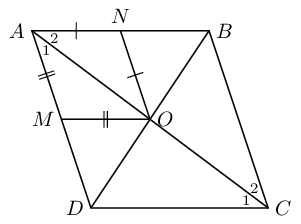In $$\triangle AMO$$ and $$\triangle ANO$$

$$\hat{A}_1 = \hat{A}_2$$ (given rhombus $$ABCD$$, diagonal $$AC$$ bisects $$\hat{A}$$)

$$\therefore \hat{A_1} = A\hat{O}M$$ ($$\angle$$s opp equal sides)

similarly $$\hat{A}_2 = A\hat{O}N$$

$$\therefore \hat{A}_2 = A\hat{O}M$$ and $$\hat{A}_1 = A\hat{O}N$$

but these are alternate interior $$\angle$$s

$$\therefore AN \parallel MO$$ and $$AM \parallel NO$$

$$\therefore ANOM$$ is a parallelogram

$$\therefore AM = NO$$ (opp sides of $$\parallel$$m)

$$\therefore AM = MO = ON = NO$$

$$\therefore ANOM$$ is a rhombus (all sides equal and two pairs of sides parallel)

$$ABCD$$ is a parallelogram with diagonal $$AC$$. Given that $$AF = HC$$, show that:

$$\triangle AFD \equiv \triangle CHB$$

\begin{align*} \hat{A}_1 & = \hat{C}_1 \qquad \text{(alt }\angle \text{s; }AD \parallel BC \text{)}\\ AD & = BC \qquad \text{(opp sides } \parallel \text{m)}\\ AF& = HC \qquad \text{(given)}\\ \therefore \triangle AFD &\equiv \triangle CHB \qquad \text{(SAS)} \end{align*}

$$DF \parallel HB$$

\begin{align*} \hat{F}_1 &= \hat{H}_1 \qquad (\triangle AFD \equiv \triangle CHB)\\ \therefore \hat{F}_1 +\hat{F}_2 &= 180° \qquad \text{(}\angle\text{s on str line)}\\ \text{and } \hat{H}_1 +\hat{H}_2 &= 180° \qquad \text{(}\angle \text{s on str line)}\\ \therefore \hat{F}_1 &= 180° - \hat{F}_2 \\ \text{and } \hat{H}_1 &= 180° - \hat{H}_2 \\ \therefore 180° - \hat{F}_2 & = 180° - \hat{H}_2 \\ \therefore \hat{F}_2 & = \hat{H}_2 \\ \therefore DF &\parallel HB \qquad \text{(corresp } \angle \text{s equal)} \end{align*}

$$DFBH$$ is a parallelogram

\begin{align*} FD & = HB \qquad (\triangle AFD \equiv \triangle CHB)\\ \text{and }DF &\parallel HB \qquad \text{ (proved above)}\\ \therefore DFBH & \text{ is a parallelogram (one pair opp sides equal and parallel)} \end{align*}

Given parallelogram $$ABCD$$ with $$AE$$ bisecting $$\hat{A}$$ and $$FC$$ bisecting $$\hat{C}$$.Write all interior angles in terms of $$y$$.

First number the angles: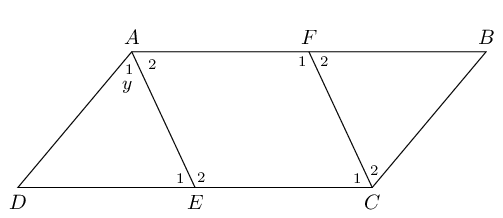Prove that $$AFCE$$ is a parallelogram.

\begin{align*} AF &\parallel EC \qquad \text{(opp sides of} \parallel \text{m)}\\ \text {and }\hat{C_1} + \hat{E_2} & = y + (180° - y)\\ \therefore & \text{ the sum of the co-interior angles is } 180°\\ \therefore AE & \parallel FC \\ \therefore AFCE & \text{ is a parallelogram (both pairs opp. sides parallel)} \end{align*}

Given that $$WZ = ZY = YX$$, $$\hat{W} = \hat{X}$$ and $$WX \parallel ZY$$, prove that: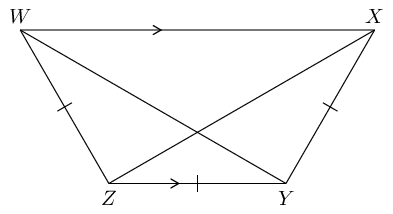$$XZ$$ bisects $$\hat{X}$$

First label the angles: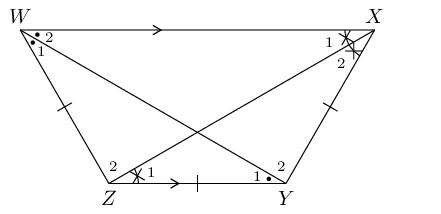$$WY = XZ$$

\begin{align*} \text{Similarly, }WY &\text{ bisects }\hat{W}\\ \therefore \hat{W_1} &= \hat{W_2}\\ \text{and }\hat{W} &= \hat{X} \text{ (given)}\\ \therefore \hat{W_1} &= \hat{W_2} = \hat{X_1} = \hat{X_2}\\ \text{ and }\hat{W_1} &= \hat{Y_1} ~(\angle \text{s opp equal sides)}\\ \text{In }\triangle WZY&\text{ and }\triangle XYZ\\ WZ&=XY \text{ (given)}\\ ZY &\text{ is a common side}\\ \hat{Z} &= \hat{Y} \text{(third }\angle \text{ in }\triangle \text{)}\\ \therefore \triangle WZY &\equiv \triangle XYZ \text{ (SAS)} \\ \therefore WY & = XZ \end{align*}

$$D$$ is a point on $$BC$$, in $$\triangle ABC$$. $$N$$ is the mid-point of $$AD$$. $$O$$ is the mid-point of $$AB$$ and $$M$$ is the mid-point of $$BD$$. $$NR \parallel AC$$.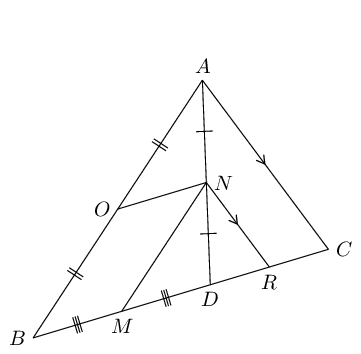Prove that $$OBMN$$ is a parallelogram.

$$AO = OB$$ (given)

$$AN = ND$$ (given)

$$\therefore ON \parallel BD$$ (Midpt Theorem)

$$BM = MD$$ (given)

$$AN = ND$$ (given)

$$\therefore MN \parallel AB$$ (Midpt Theorem)

$$\therefore OBMN$$ is a parallelogram (both pairs opp. sides parallel)

Prove that $$BC = 2MR$$.

$$AN = NC$$ (given)

$$NR \parallel AC$$ (given)

$$\therefore DR = RC$$ (Midpt Theorem)

$$\therefore DR = \frac{1}{2} DC$$

$$MD = \frac{1}{2}BD$$ (given)

$$\therefore MD + DR = \frac{1}{2} (BD + DC)$$

$$MR = \frac{1}{2}BC$$

$$\therefore BC = 2MR$$

In $$\triangle MNP$$, $$\hat{M} = 90°$$, $$S$$ is the mid-point of $$MN$$ and $$T$$ is the mid-point of $$NR$$.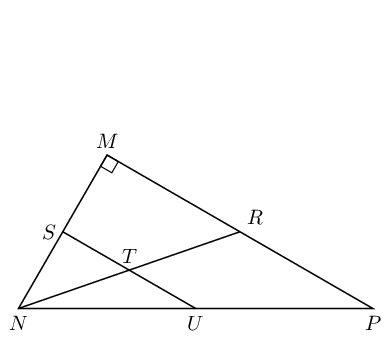Prove $$U$$ is the mid-point of $$NP$$.

\begin{align*} NS&=SM \text{ (given)}\\ NT&=TR \text{ (given)}\\ \therefore ST &\parallel MR \text{ (Midpt Theorem)} \\ \therefore U & \text{ is the mid-point of } NP \text{ (converse of Midpt Theorem)} \end{align*}

If $$ST = \text{4}\text{ cm}$$ and the area of $$\triangle SNT$$ is $$\text{6}$$ $$\text{cm^{2}}$$, calculate the area of $$\triangle MNR$$.

\begin{align*} N\hat{S}T & = 90° \text{ (corresp }\angle \text{s; } ST \parallel MR)\\ \therefore \text{ area }\triangle SNT &=\frac{1}{2} ST \times SN \\ 6 &= \frac{1}{2}(4)SN\\ \therefore SN &= \text{3}\text{ cm}\\ \therefore MN &= \text{6}\text{ cm} \\ MR &= 2ST = \text{8}\text{ cm}\\ \text{area }\triangle MNR &= \frac{1}{2} MR \times MN\\ &=\frac{1}{2} (8)(6)\\ &= \text{24}\text{ cm$^{2}$} \end{align*}

Prove that the area of $$\triangle MNR$$ will always be four times the area of $$\triangle SNT$$, let $$ST = x \text{ units}$$ and $$SN = y \text{ units}$$.

\begin{align*} \text{Let }ST \text{ be } & x \text{ units}\\ \therefore MR \text{ will be } & 2x\\ \text{Let }SN \text{ be } & y \text{ units}\\ \therefore MN \text{ will be } & 2y\\ \text{area }\triangle SNT & = \frac{1}{2}xy \\ \text{area }\triangle MNR & = \frac{1}{2} (2x)(2y) \\ & = 2xy\\ \therefore \text{ area } \triangle MNR &= 4\left(\frac{1}{2}xy\right) \\ & = 4(\text{area }\triangle SNT) \end{align*}

Given quadrilateral $$QRST$$ with sides $$QR \parallel TS$$ and $$QT \parallel RS$$. Also given: $$\hat{Q} = y$$ and $$\hat{S} = 63^{\circ}$$; $$Q\hat{T}R = 38^{\circ}$$ and $$R\hat{T}S = x$$. Complete the proof below to prove that $$QRST$$ is a parallelogram.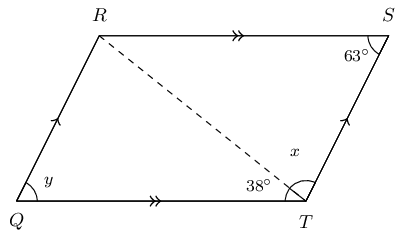The completed proof looks like this:

$\begin{array}{|l | l|} \hline \text{Steps} & \text{Reasons} \\ \hline Q\hat{T}R = T\hat{R}S & \text{alt } \angle \text{s; } QT \parallel RS \\ S\hat{T}R = Q\hat{R}T & \text{alt } \angle \text{s; } QR \parallel TS \\ \text{In } \triangle QRT \text{ and } \triangle RST \text{ side } RT = RT & \text{ common side } \\ \therefore \triangle QRT \equiv \triangle STR &\text{congruent (AAS)} \\ \therefore QR = TS \text{ and } QT = RS & \text{congruent triangles} \\ \hat{Q} = \hat{S} & \text{congruent triangles} \\ \therefore QRST \text{ is a parallelogram} &\text{opp sides of quad are } = \\ \hline \end{array}$

Calculate the value of $$y$$.

$$QRST$$ is a parallelogram, $$\therefore \hat{Q} = \hat{S}$$.

Opposite $$\angle$$s of parallelogram are equal. $$\hat{Q} = \hat{S}$$ and $$\hat{R} = \hat{T}$$.

Therefore, $$y = 63^{\circ}$$.

Calculate the value of $$x$$.

$$\angle$$s in a $$\triangle = 180 ^{\circ}$$

$$\therefore \hat{Q} + Q\hat{R}T + Q\hat{T}R = 180 ^{\circ}$$

Now we know that $$\hat{Q} = \hat{S} = 63^{\circ}$$ and that $$R\hat{T}S = 79^{\circ}$$.

$$\therefore \hat{x} = 180^{\circ} - 63^{\circ} - 79^{\circ} = 79^{\circ}$$.

Study the quadrilateral $$QRST$$ with opposite angles $$\hat{Q} = \hat{S} = 117^{\circ}$$ and angles $$\hat{R} = \hat{T} = 63^{\circ}$$ carefully. Fill in the correct reasons or steps to prove that the quadrilateral $$QRST$$ is a parallelogram.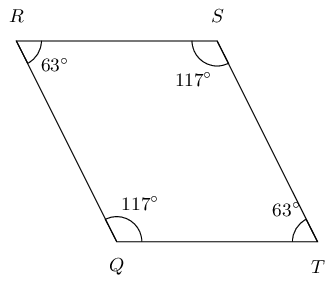$\begin{array}{|l |l|} \hline \text{Steps} & \text{Reasons} \\ \hline R\hat{Q}T = R\hat{S}T & \text{given both } \angle \text{s } = 117^\circ \\ Q\hat{R}S = Q\hat{T}S & \text{given both } \angle \text{s } = 63^\circ \\ \hat{Q} + \hat{R} + \hat{S} + \hat{T} = 360 ^\circ & \text{sum of } \angle \text{s in quad} \\ R\hat{Q}T + Q\hat{T}S = 180 ^\circ & 117^\circ + 63^\circ = 180^\circ \\ \therefore QR \parallel TS & \text{co-int } \angle \text{s; } QR \parallel TS \\ \therefore RS \parallel QT & \text{co-int } \angle \text{s; } RS \parallel QT \\ \therefore QRST \text{ is a parallelogram} & \text{opp. sides parallel} \\ \hline \end{array}$

Study the quadrilateral $$QRST$$ with $$\hat{Q} = \hat{S} = 149^{\circ}$$ and $$\hat{R} = \hat{T} = 31^{\circ}$$ carefully. Fill in the correct reasons or steps to prove that the quadrilateral $$QRST$$ is a parallelogram.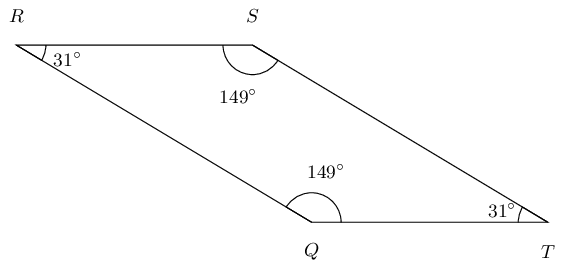$\begin{array}{|l |l|} \hline \text{Steps} & \text{Reasons} \\ \hline R\hat{Q}T = R\hat{S}T & \text{given both } \angle \text{s} = 149^{\circ} \\ Q\hat{R}S = Q\hat{T}S & \text{given both } \angle \text{s} = 31^{\circ} \\ \hat{Q} + \hat{R} + \hat{S} + \hat{T} = 360 ^{\circ} & \text{sum of } \angle \text{s in quad} \\ R\hat{Q}T + Q\hat{T}S = 180 ^{\circ} & 149^{\circ} + 31^{\circ} = 180^{\circ} \\ \therefore QR \parallel TS & \text{co-int } \angle \text{s; } QR \parallel TS \\ \therefore RS \parallel QT & \text{co-int } \angle \text{s; } RS \parallel QT \\ \therefore QRST \text{ is a parallelogram} & \text{opp. sides parallel} \\ \hline \end{array}$

In parallelogram $$QTRS$$, the bisectors of the angles have been constructed, indicated with the red lines below. You are also given $$QT = SR$$, $$TR = QS$$, $$QT \parallel SR$$, $$TR \parallel QS$$, $$\hat{Q} = \hat{R}$$ and $$\hat{T} = \hat{S}$$.

Prove that the quadrilateral $$JKLM$$ is a parallelogram.

Note the diagram is drawn to scale.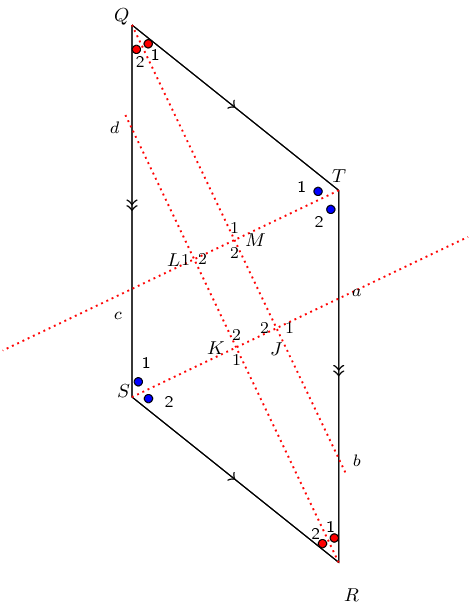Redraw the diagram and mark all the known information: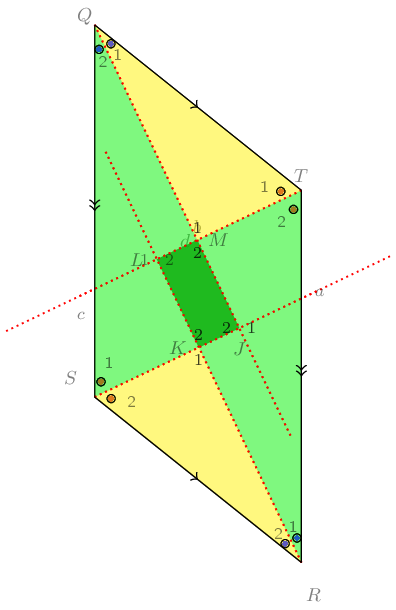Study the diagram below; it is not necessarily drawn to scale. Two triangles in the figure are congruent: $$\triangle CDE \equiv \triangle CBF$$. Additionally, $$EA = ED$$. You need to prove that $$ABFE$$ is a parallelogram.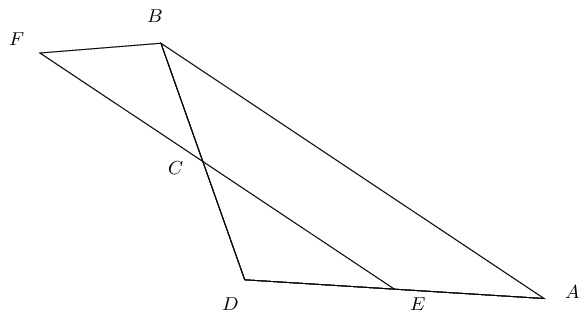Redraw the diagram and mark all known and given information: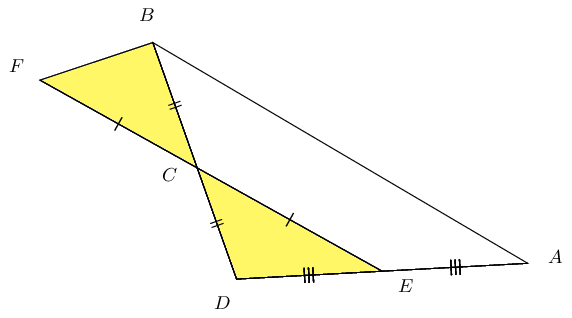Given the following diagram: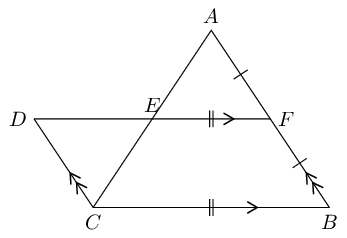Show that $$BCDF$$ is a parallelogram.

\begin{align*} DF & \parallel CB \text{ (given)} \\ DC & \parallel FB \text{ (given)} \\ \therefore BCDF & \text{ is a parallelogram (both pairs opp. sides } \parallel \text{)} \end{align*}

Show that $$ADCF$$ is a parallelogram.

\begin{align*} \text{In } \triangle DEC & \text{ and } \triangle FEA \\ C\hat{A}F & = A\hat{C}D \text{ (alt } \angle\text{s; } AB \parallel DC \text{)} \\ A\hat{F}D & = C\hat{D}F \text{ (alt } \angle\text{s; } AB \parallel DC \text{)} \\ DC & = FB \text{ (opp sides parm eq)} \\ \therefore DC & = FA = FB \\ \therefore \triangle DEC & \equiv \triangle FEA \text{ (ASA)} \\ \therefore DE = EF & \text{ and } CE = EA \end{align*}

But $$AE$$ and $$DF$$ are diagonals of $$ADCF$$, $$\therefore ADCF$$ is a parallelogram (diagonals bisect each other).

Prove that $$AE = EC$$.

$$AE = EC$$ (proved above).

$$ABCD$$ is a parallelogram. $$BEFC$$ is a parallelogram. $$ADEF$$ is a straight line. Prove that $$AE = DF$$.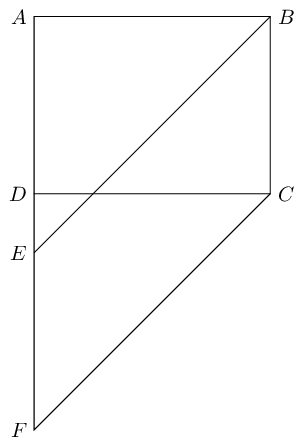\begin{align*} BC & = EF \text{ (opp sides of } \parallel \text{m)} \\ BC & = AD \text{ (opp sides of } \parallel \text{m)} \\ \therefore EF & = ED \\ AD + DE & = AE \\ EF + DE & = DF \\ \text{ but } DE & \text{ is common} \\ \therefore AE & = DF \end{align*}

In the figure below $$AB = BF$$, $$AD = DE$$. $$ABCD$$ is a parallelogram. Prove $$EF$$ is a straight line.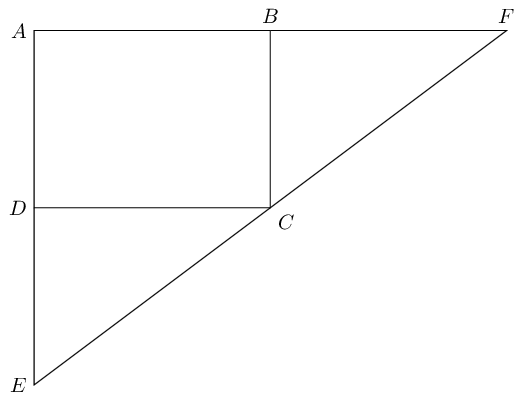We note that:

\begin{align*} B\hat{A}D & = B\hat{C}D \text{ (opp } \angle\text{s } \parallel \text{m)} \\ C\hat{D}E & = B\hat{C}D \text{ (alt } \angle\text{s; } AE \parallel BC \text{)} \\ F\hat{B}C & = B\hat{C}D \text{ (alt } \angle\text{s; } AF \parallel DC \text{)} \\ \therefore C\hat{D}E & = F\hat{B}C \end{align*}

We also note that:

\begin{align*} AD & = BC \text{ (opp sides parm eq)} \\ AB & = DC \text{ (opp sides parm eq)} \end{align*}

Now we can show that $$\triangle DEC$$ is congruent to $$\triangle BCF$$:

\begin{align*} \text{in } \triangle DEC & \text{ and } \triangle BCF\\ C\hat{D}E & = F\hat{B}C \qquad \text{(proven above)} \\ DC = AB & = BF \qquad \text{(given)} \\ DE = AD & = BC \qquad \text{(given)} \\ \therefore \triangle DEC & \equiv \triangle BCF \text{ (SAS)} \end{align*}

Finally we can show that $$ECF$$ is a straight line:

\begin{align*} \therefore B\hat{F}C & = D\hat{C}E \text{ (}\triangle DEC \equiv \triangle BCF \text{)} \\ B\hat{C}F & = D\hat{E}C \text{ (}\triangle DEC \equiv \triangle BCF \text{)} \\ \text{but } F\hat{B}C + B\hat{F}C + B\hat{C}F & = 180° \text{(sum of }\angle\text{s in } \triangle \text{)} \\ \therefore D\hat{C}E + B\hat{C}F + B\hat{C}D & = 180° \\ \therefore ECF & \text{ is a str line} \end{align*}